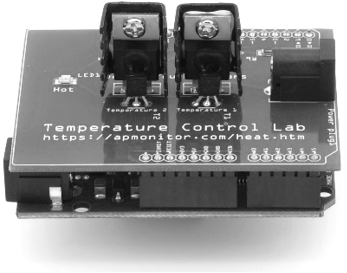## Quiz on PID Control1. If the set point is constant (\frac{dSP(t)}{dt}=0) which of the following is equivalent to \frac{de(t)}{dt}?

A. -\frac{dPV}{dt}
Correct. \frac{de(t)}{dt} = \frac{dSP(t)}{dt} - \frac{dPV(t)}{dt} = - \frac{dPV(t)}{dt}
B. \frac{dPV}{dt}
Incorrect. \frac{de(t)}{dt} = \frac{dSP(t)}{dt} - \frac{dPV(t)}{dt} = - \frac{dPV(t)}{dt}
C. \frac{dSP}{dt}
Incorrect. \frac{de(t)}{dt} = \frac{dSP(t)}{dt} - \frac{dPV(t)}{dt} = - \frac{dPV(t)}{dt}
D. 0
Incorrect. \frac{de(t)}{dt} = \frac{dSP(t)}{dt} - \frac{dPV(t)}{dt} = - \frac{dPV(t)}{dt}

2. What is the advantage of using the replacement for \frac{de(t)}{dt} from question 1?

A. It is a simple reformulation with no particular advantage
Incorrect. It removes derivative kicks, removing unnecessary stress on valves
B. Removing derivative kicks has the effect of significantly improving the overall operation of the system
Incorrect. The change to remove derivative kicks usually has little impact on the overall performance of the system, but can harm a valve or similar part that the actuator acts on
C. It will reduce potential wear/stress on valves by removing derivative kicks
Correct. A derivative kick typically sends the actuator to an upper or lower limit for one controller cycle. It typically has little effect on the process but can wear out a valve or other equipment.
D. It simplifies the equation.
Incorrect. It removes derivative kicks when there are set point changes, removing unnecessary stress on valves or other actuators.

3. What are the simple tuning correlations (IMC with \theta_p=0)? Try to recall this without referencing the page. Choose from the options below.

A. K_c = K_p, tau_I = \tau_p, \tau_D = 0
B. K_c = \frac{1}{K_p}, \tau_I = \tau_p, tau_D = 0
Correct. Correct
C. K_c = \frac{1}{K_p}, \tau_I = 0, \tau_D = \tau_p
D. K_c = K_p, \tau_I = 0, \tau_D = \tau_p
4. What is the value of the derivative filter constant \alpha with moderate tuning and negligible dead time \theta_p?
Incorrect. The value approaches \frac{\tau_p^2}{\tau_p^2} which is 1.
Incorrect. The value approaches \frac{\tau_p^2}{\tau_p^2} which is 1.
C. \tau_p
Incorrect. The value approaches \frac{\tau_p^2}{\tau_p^2} which is 1.
Correct. The value approaches \frac{\tau_p^2}{\tau_p^2}